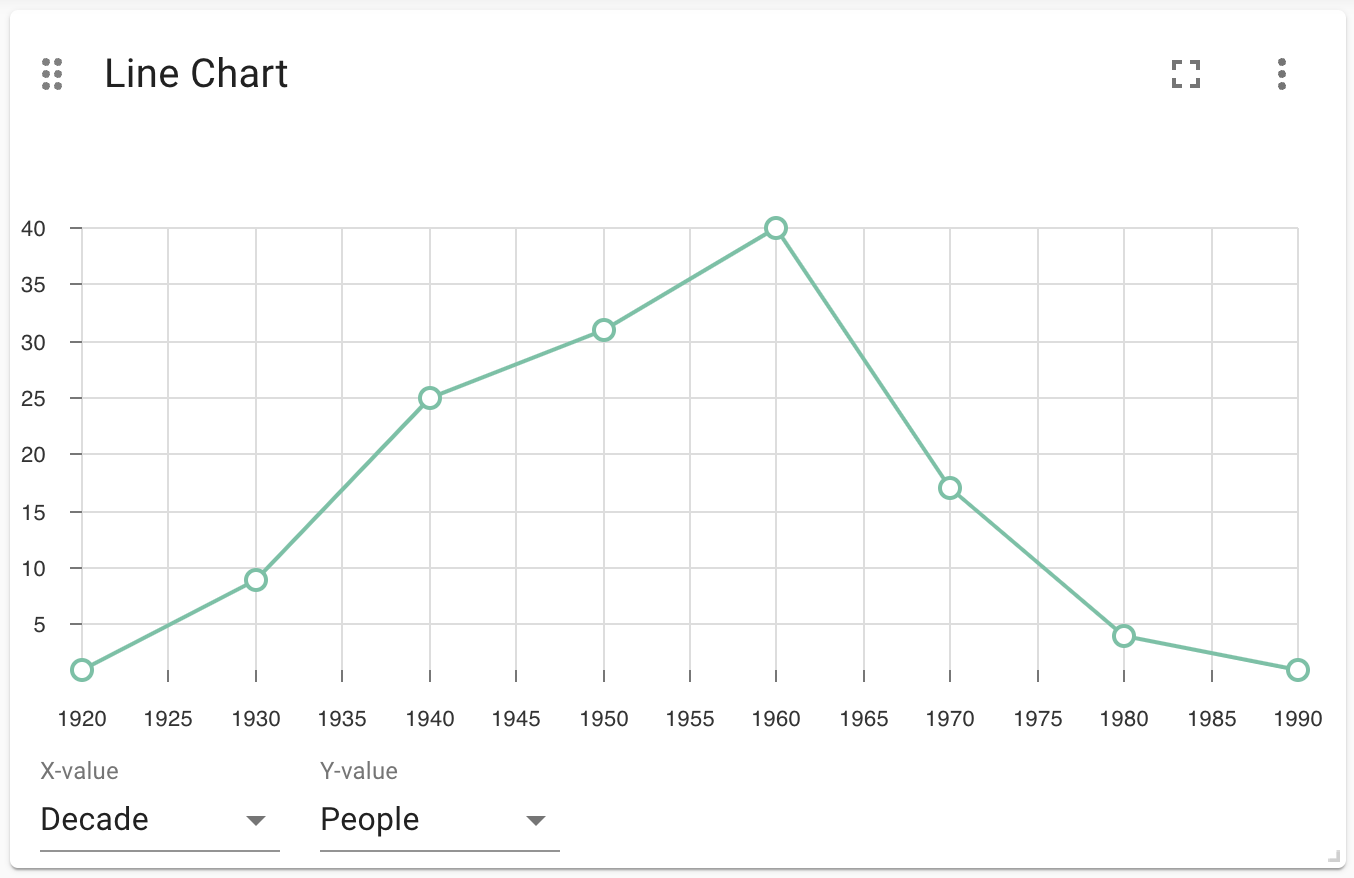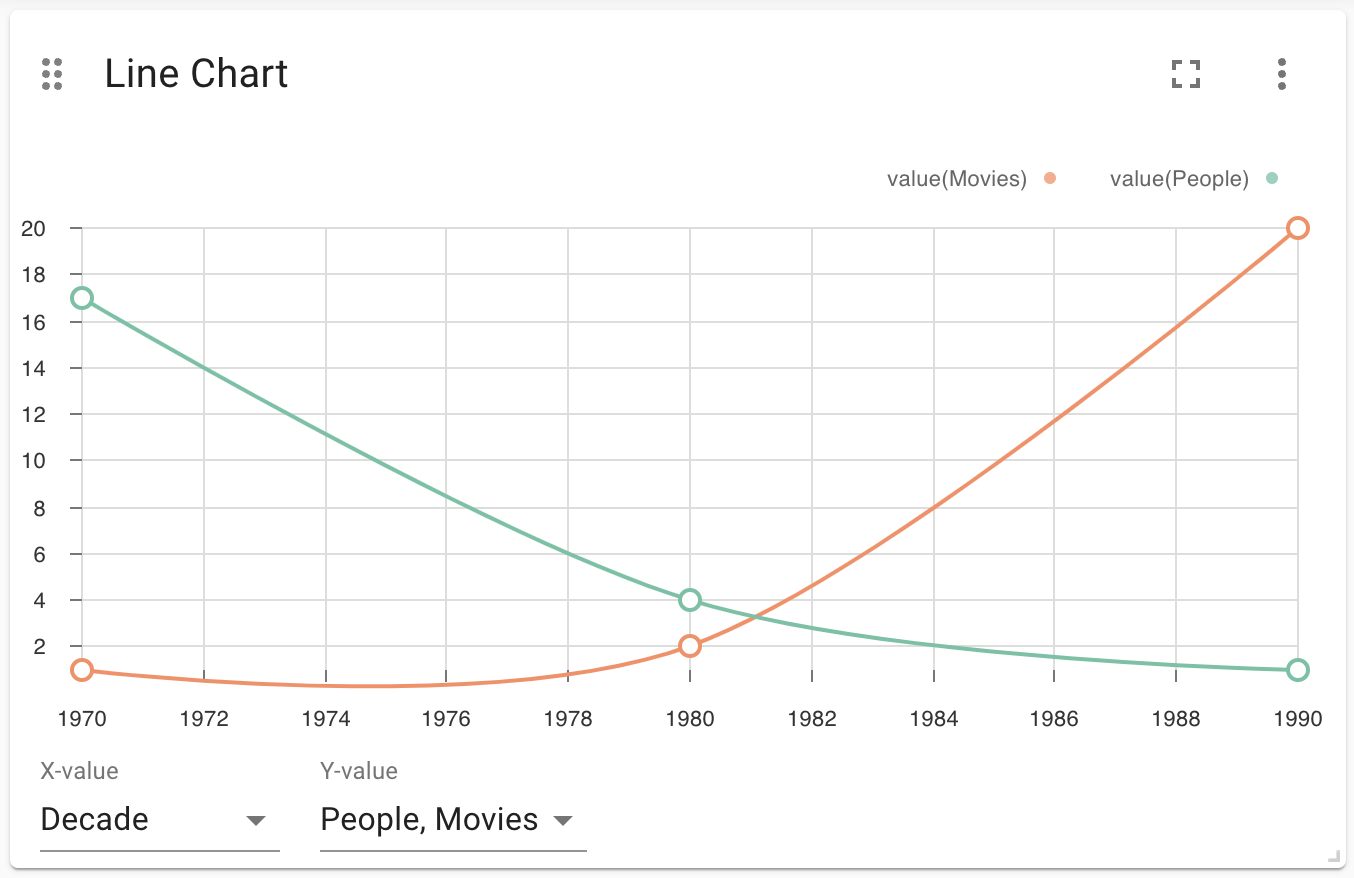# Line Chart

A line chart can be used to draw one or more lines in a two-dimensional plane. It requires two numeric fields:

• X-value: a numeric field. These will be the values used as an X-coordinate.

• Y-Value: a numeric field. These will be the values used as an Y-coordinate.

Always ensure that the X-value are sorted in ascending order. If not, the chart will not be displayed correctly.

The line chart supports plotting both simple numbers and time values on the x-axis. If you select a Neo4j datetime property on the x-axis, the chart will automatically be drawn as a time series.

## Examples

### Basic Line Chart (Actors born by decade)

```MATCH (p:Person)
RETURN  (p.born/10)*10 as Decade, COUNT(p) as People### Multi-Line Chart (Actors born & movies released by decade)

```MATCH (p:Person)
WITH  (p.born/10)*10 as Decade, COUNT(p) as People
MATCH (m:Movie)
RETURN Decade, People, COUNT(DISTINCT m) as Movies```Name Type Default Value Description

Show Legend

on/off

off

If enabled, shows a legend at the top right of the visualization.

Color Scheme

List

neodash

The color scheme to use for the lines. Colors are assigned automatically to the different fields selected in the report footer.

X Scale

List

linear

How to scale the values on the x-axis. Can be either linear, logarithmic or point. Use point for categorical data.

Y Scale

List

linear

How to scale the values on the y-axis. Can be either linear or logarithmic.

Min X Value

Number

auto

If not set to ``auto'', this variable is the minimum value on the x-axis.

Max X Value

Number

auto

If not set to ``auto'', this variable is the maximum value on the x-axis.

Min Y Value

Number

auto

If not set to ``auto'', this variable is the minimum value on the y-axis.

Max Y Value

Number

auto

If not set to ``auto'', this variable is the maximum value on the y-axis.

X-axis Tick Count

Number

auto

If not set to ``auto'', the number of ticks to be made on the x-axis. This is an approximate number that the visualization tries to adhere to (numeric X-axis only)

X-axis Format (Time chart)

Text

%Y-%m-%dT%H:%M:%SZ

When using a time chart, this setting lets you override how time values are rendered on the x-axis. This uses the ISO 8601 time notations.

X-axis Tick Size (Time chart)

Text

every 1 year

When using a time chart, this setting helps you set the frequency of ticks. The text format should look like this: `"every [number] ['years', 'months', 'weeks', 'days', 'hours', 'seconds', 'milliseconds']"`.

Line Smoothing

List

linear

Determines how the lines in the chart are smoothened. One of linear (no smoothing), basis (interpolating), cardinal (through each point) and step (step-based interpolation).

X-axis Tick Rotation (Degrees)

number

0

The angle at which the tick labels on the x-axis are rotated.

Y-axis Tick Rotation (Degrees)

number

0

The angle at which the tick labels on the y-axis are rotated.

Show Grid

on/off

on

If enabled, shows a grid in the line chart that intersects at the axis ticks.

number

10

The size of a point on each line.

Line Width (px)

number

2

The width (in pixels) of each line in the chart.

Margin Left (px)

number

50

The margin in pixels on the left side of the visualization.

Margin Right (px)

number

24

The margin in pixels on the right side of the visualization.

Margin Top (px)

number

24

The margin in pixels on the top side of the visualization.

Margin Bottom (px)

number

40

The margin in pixels on the bottom side of the visualization.

Legend Width (px)

number

128

The width in pixels of each legend label on top of the visualization (if enabled).

Hide Property Selection

on/off

off

If enabled, hides the property selector (footer of the visualization).

Auto-run query

on/off

on

when activated automatically runs the query when the report is displayed. When set to `off', the query is displayed and will need to be executed manually.

Report Description

markdown text

When specified, adds another button the report header that opens a pop-up. This pop-up contains the rendered markdown from this setting.

## Rule-Based Styling

Using the Rule-Based Styling menu, the following style rules can be applied to the line chart:

• The color of the line.